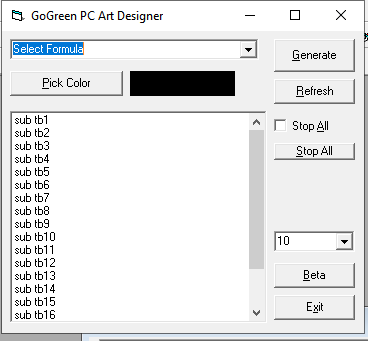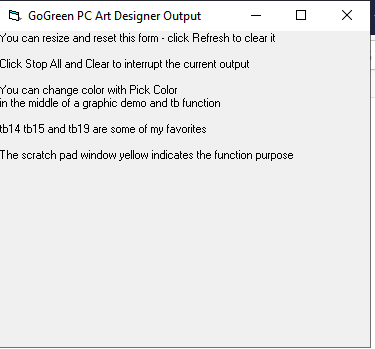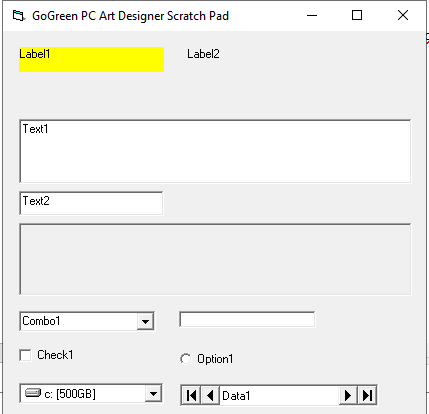VB6 mathematics and color examples - sample code

by Mountain Computers Inc., Publication Date: Sunday, October 6, 2019View Count: 450, Keywords: VB6, Art, Math, Trig, Colors, Hashtags: #VB6 #Art #Math #Trig #Colors

This is a freebie to those that want to play with graphics and mathematics and timers and some degree of logic and looping, and colors and APIs -- those that like to play with trigonometry and colors and code samples.

This has nothing to do with the Go Green PC Tune Up™

This has to do a few days of playing with vb6 coding colors and math and some output and screen management. Remember, you can create something like this in C# and VB.Net not just Visual Basic 6 Professional.

Have fun and enjoy. The screen shots help with the UI.

Link to the ZIP file with the code sample and the EXE that you can run right away and play.'used with permission from andrew flagg

Option Explicit
Private Declare Sub Sleep Lib "kernel32" (ByVal dwMilliseconds As Long)

'colorstruct

Private Type ChooseColorStruct
lStructSize As Long
hwndOwner As Long
hInstance As Long
rgbResult As Long
lpCustColors As Long
flags As Long
lCustData As Long
lpfnHook As Long
lpTemplateName As String
End Type

Private Declare Function ChooseColor Lib "comdlg32.dll" Alias "ChooseColorA" _
(lpChoosecolor As ChooseColorStruct) As Long
Private Declare Function OleTranslateColor Lib "oleaut32.dll" (ByVal lOleColor _
As Long, ByVal lHPalette As Long, lColorRef As Long) As Long

Private Const CC_RGBINIT = &H1&
Private Const CC_FULLOPEN = &H2&
Private Const CC_PREVENTFULLOPEN = &H4&
Private Const CC_SHOWHELP = &H8&
Private Const CC_ENABLEHOOK = &H10&
Private Const CC_ENABLETEMPLATE = &H20&
Private Const CC_ENABLETEMPLATEHANDLE = &H40&
Private Const CC_SOLIDCOLOR = &H80&
Private Const CC_ANYCOLOR = &H100&
Private Const CLR_INVALID = &HFFFF

Private Sub BtnBeta_Click()

'SC.Top = GG.Top + GG.Height
'SC.Left = GG.Left
'Call realign_windows
'Call t15
End Sub

Private Sub BtnGenerate_Click()
If HH.Combo1.Text = "Select Formula" Then
Else
Select Case HH.Combo1.Text
Case "kscope"

Case Else
End Select
End If
End Sub

Private Sub BtnPickColor_Click()
Dim b
b = ShowColorDialog
Debug.Print b
HH.Shape1.FillStyle = 0
HH.Shape1.FillColor = b
'HH.Picture1.Refresh
End Sub

Private Sub BtnRefresh_Click()
Call realign_windows
HH.ChkStopAll.Value = 0
HH.BtnStopAll.Caption = "&Stop All"
End Sub

Private Sub BtnStopAll_Click()
If HH.ChkStopAll.Value = 0 Then
HH.ChkStopAll.Value = 1
HH.BtnStopAll.Caption = "&Clear"
Else
HH.ChkStopAll.Value = 0
HH.BtnStopAll.Caption = "&Stop All"
End If
End Sub

Me.Combo1.Text = "Select Formula"
o.Clear

Me.Shape1.FillStyle = 0
Me.Shape1.FillColor = vbBlack

x1 = GG.Left
y1 = GG.Top
x2 = GG.Width
y2 = GG.Height

Call realign_windows
Call setTimerDelay

GG.Print "You can resize and reset this form - click Refresh to clear it"
GG.Print
GG.Print "Click Stop All and Clear to interrupt the current output"
GG.Print
GG.Print "You can change color with Pick Color"
GG.Print "in the middle of a graphic demo and tb function"
GG.Print
GG.Print "tb14 tb15 and tb19 are some of my favorites"
GG.Print
GG.Print "The scratch pad window yellow indicates the function purpose"

End Sub

Private Sub BtnExit_Click()
End
End Sub
Private Sub o_DblClick()
'call function
Dim c

Call realign_windows

If Left(o.Text, Len("sub tb")) = "sub tb" Then
c = Val(Right(o.Text, Len(o.Text) - Len("sub tb")))
'Debug.Print c
Select Case c

Case 1
Call t1
Case 2
Call t2
Case 3
Call t3
Case 4
Call t4
Case 5
Call t5
Case 6
Call t6
Case 7
Call t7
Case 8
Call t8
Case 9
Call t9
Case 10
Call t10
Case 11
Call t11
Case 12
Call t12
Case 13
Call t13
Case 14
Call t14
Case 15
Call t15
Case 16
Call t16
Case 17
Call t17
Case 18
Call t18
Case 19
Call t19
Case 20
Call t20
Case Else

End Select

End If

End Sub

Sub realign_windows()
Debug.Print "realign_windows()"
GG.Left = HH.Left + HH.Width
GG.Top = HH.Top
GG.Show
GG.Refresh
SC.Left = GG.Left
SC.Top = GG.Top + GG.Height
SC.Show
SC.Refresh
End Sub

'function tb1-?
Dim v
v = 0
For v = 1 To 20
Next

End Sub

End

End Sub

Sub t1()
SC.Label1 = "create line, randomize"
Dim x, Y

Randomize
Debug.Print Rnd

While x < 1000
x = x + 1
Y = Y + 1
GG.PSet (x, Y), HH.Shape1.FillColor
Wend

End Sub

Sub t2()

SC.Label1 = "create circle"
GG.ScaleMode = 1
GG.Circle (ScaleWidth / 2, ScaleHeight / 2), Switch(ScaleWidth >= ScaleHeight, ScaleHeight / 2, ScaleWidth < ScaleHeight, ScaleWidth / 2)

End Sub

Sub t3()

SC.Label1 = "say hello in window and picture"
SC.CurrentX = ScaleWidth / 4
SC.CurrentY = ScaleHeight / 4
SC.FontBold = True
SC.FontSize = 12
SC.Print "Hello from Visual Basic"

SC.Picture1.CurrentX = SC.Picture1.ScaleWidth / 4
SC.Picture1.CurrentY = SC.Picture1.ScaleHeight / 4
SC.Picture1.FontSize = 12
SC.Picture1.Print "Hello from Visual Basic"

End Sub
Sub t4()

SC.Label1.Caption = "list fonts, doevents()"

'this can be a long one

Dim x
x = 0

Dim intLoopIndex As Integer

For intLoopIndex = 0 To Screen.FontCount
SC.Text1.Text = x & "). " & Screen.Fonts(intLoopIndex) & vbCrLf & SC.Text1.Text
x = x + 1
SC.Refresh
DoEvents
If ChkStopAll.Value = 1 Then GoTo out
Next intLoopIndex

out:

End Sub

Sub t5()

SC.Label1 = "increase text1 as new font object"
Dim Font1 As New StdFont
Font1.Size = 24
Font1.Name = "Arial"
Set SC.Text1.Font = Font1

End Sub

Sub t6()

SC.Label1 = "create crossed lines"

GG.Line (0, 0)-(ScaleWidth, ScaleHeight)
GG.Line (ScaleWidth, 0)-(0, ScaleHeight)

SC.Picture1.Line (0, 0)-(SC.Picture1.ScaleWidth, SC.Picture1.ScaleHeight)
SC.Picture1.Line (SC.Picture1.ScaleWidth, 0)-(0, SC.Picture1.ScaleHeight)

End Sub

Sub t7()
SC.Label1 = "create line"
GG.Line (ScaleWidth / 2, ScaleHeight / 2)-(2 * ScaleWidth / 2, 2 * ScaleHeight / 2)

End Sub

Sub t8()

SC.Label1 = "create box in window and picture"

GG.DrawStyle = vbDash
GG.Line (ScaleWidth / 7, ScaleHeight / 4)-(1.4 * ScaleWidth / 6, 2 * ScaleHeight / 4)

GG.DrawStyle = vbSolid
GG.FillColor = RGB(255, 0, 0)
GG.FillStyle = vbFSSolid
GG.Line (ScaleWidth / 4, ScaleHeight / 4)-(3 * ScaleWidth / 4, 3 * ScaleHeight / 4)

'FF0000 blue, 0000FF red,
SC.Show
SC.Picture1.FillColor = &HFF0000
SC.Picture1.FillStyle = 0
SC.Picture1.Line (SC.Picture1.ScaleWidth / 4, SC.Picture1.ScaleHeight / 4)-(3 * SC.Picture1.ScaleWidth / 4, 3 * SC.Picture1.ScaleHeight / 4)

End Sub

Sub t9()
SC.Label1 = "create elipse in window and picture"
GG.Circle (ScaleWidth / 2, ScaleHeight / 2), Switch(ScaleWidth >= ScaleHeight, ScaleHeight / 2, ScaleWidth < ScaleHeight, ScaleWidth / 2), &HFF, , , 0.6
SC.Picture1.Circle (SC.Picture1.ScaleWidth / 2, SC.Picture1.ScaleHeight / 2), Switch(SC.Picture1.ScaleWidth >= SC.Picture1.ScaleHeight, SC.Picture1.ScaleHeight / 2, SC.Picture1.ScaleWidth < _
SC.Picture1.ScaleHeight, SC.Picture1.ScaleWidth / 2), &HFF0000, , , 0.6

End Sub

Sub t10()
SC.Label1 = "create arc in window and picture"
GG.Circle (ScaleWidth / 2, ScaleHeight / 2), Switch(ScaleWidth >= ScaleHeight, ScaleHeight / 2, ScaleWidth < ScaleHeight, ScaleWidth / 2), &HFF, , 2.14, 0.6
SC.Picture1.Circle (SC.Picture1.ScaleWidth / 2, SC.Picture1.ScaleHeight / 2), Switch(SC.Picture1.ScaleWidth >= SC.Picture1.ScaleHeight, SC.Picture1.ScaleHeight / 2, SC.Picture1.ScaleWidth < _
SC.Picture1.ScaleHeight, SC.Picture1.ScaleWidth / 2), &HFF0000, , 2.14, 0.6
End Sub

Sub t11()

Dim intLoopIndex As Integer
For intLoopIndex = 1 To 9
DrawWidth = intLoopIndex
GG.Line (0, intLoopIndex * ScaleHeight / 10)-(ScaleWidth, intLoopIndex * ScaleHeight / 10)
If ChkStopAll.Value = 1 Then GoTo out
Next intLoopIndex
out:
DrawMode = vbInvert
DrawWidth = 10
GG.Line (0, 0)-(ScaleWidth, ScaleHeight)
GG.Line (0, ScaleHeight)-(ScaleWidth, 0)

End Sub

Sub t12()
SC.Label1 = "show print array"
Dim prt As Printer
GG.Print "** LIST OF PRINTERS ON YOUR COMPUTER **"
For Each prt In Printers
GG.Print prt.DeviceName
Debug.Print prt.DeviceName
Next

Debug.Print Printer.DeviceName
End Sub

Sub t13()
SC.Label1 = "create mesh in window"

x1 = 1
y1 = 1
x2 = 1
y2 = 1

Dim s
's step

For s = 2 To 10

GG.Refresh
GG.ScaleMode = vbPixels

For y1 = 1 To GG.ScaleHeight Step s

GG.Line (1, y1)-(GG.ScaleWidth, GG.ScaleHeight - y1), HH.Shape1.FillColor
DoEvents
If ChkStopAll.Value = 1 Then GoTo out

Next y1

For x1 = 1 To GG.ScaleWidth Step s

GG.Line (x1, 1)-(GG.ScaleWidth - x1, GG.ScaleHeight), HH.Shape1.FillColor
DoEvents
If ChkStopAll.Value = 1 Then GoTo out
Next x1

Sleep 1000

Next s
out:

End Sub

Sub t14()
SC.Label1 = "create random lines in window"

ScaleMode = vbPixels
'initial starting points for x and y
GG.Refresh
Dim l
For l = 1 To 100
Randomize
x1 = Rnd * GG.Width
y1 = Rnd * GG.Height
Randomize
x2 = Rnd * GG.Width
y2 = Rnd * GG.Height

'Debug.Print l & ") (" & x1 & ", " & y1 & "),(" & x2 & ", " & y2 & ")"
GG.Line (x1, y1)-(x2, y2), HH.Shape1.FillColor

DoEvents
Next

End Sub

Sub t15()
SC.Label1 = "create random bouncing rays in window"
ScaleMode = vbTwips
'initial starting points for x and y
GG.Refresh
Randomize
x1 = Rnd * GG.Width
y1 = Rnd * GG.Height
Randomize
x2 = Rnd * GG.Width
y2 = Rnd * GG.Height

'Debug.Print "initial line " & GG.Width & " : " & x1 & ", " & GG.Height & " : " & y1

'draw initial line
GG.Line (x1, y1)-(x2, y2), HH.Shape1.FillColor
DoEvents

'prepare to move it down and to the right until it hits boundaries

Dim l, s
Dim dx1, dy1, dx2, dy2

's = step, not yet working
'l = loop
l = 0
s = 50

dx1 = s
dy1 = s

dx2 = s
dy2 = s

For l = 1 To 5000

'lets get a direction

'extent of right and bottom boundaries
If x1 > GG.Width Then
dx1 = -s
End If

If x2 > GG.Width Then
dx2 = -s

End If

If y1 > GG.Height Then
dy1 = -s
End If

If y2 > GG.Height Then
dy2 = -s
End If

'extent of top and left boundaries
If x1 < 1 Then
dx1 = s
End If

If x2 < 1 Then
dx2 = s
End If

If y1 < 1 Then
dy1 = s
End If

If y2 < 1 Then
dy2 = s
End If

x1 = Round(x1 + dx1, 0)
x2 = Round(x2 + dx2, 0)
y1 = Round(y1 + dy1, 0)
y2 = Round(y2 + dy2, 0)

'Debug.Print l & ") (" & x1 & ", " & y1 & "),(" & x2 & ", " & y2 & ")"
GG.Line (x1, y1)-(x2, y2), HH.Shape1.FillColor

If Val(TimerDelay.Text) > 0 Then
Sleep Val(TimerDelay.Text)
End If

DoEvents
If HH.ChkStopAll.Value = 1 Then GoTo out
Next l

out:

End Sub
Sub t16()
SC.Label1 = "sine waves"
'draw a sine wave
Dim x, Y
x = 1

While x < GG.Width
DoEvents
'Debug.Print x & ", " & Y
Y = (Sin(x) * 1500) + GG.Height / 2
'GG.Line (x, y)-(x, y), HH.Shape1.FillColor
GG.PSet (x, Y), HH.Shape1.FillColor
x = x + 1
If ChkStopAll.Value = 1 Then GoTo out
Wend

out:

End Sub

Sub t17()
SC.Label1 = "cosine waves"
'draw a sine wave
Dim x, Y
x = 1

While x < GG.Width
DoEvents
'Debug.Print x & ", " & Y
Y = (Cos(x) * 1500) + GG.Height / 2
'GG.Line (x, y)-(x, y), HH.Shape1.FillColor
GG.PSet (x, Y), HH.Shape1.FillColor
x = x + 1
If ChkStopAll.Value = 1 Then GoTo out
Wend

out:

End Sub

Sub t18()

SC.Label1 = "tangent waves"
'draw a sine wave
Dim x, Y
x = 1

While x < GG.Width
DoEvents
'Debug.Print x & ", " & Y
Y = (Tan(x) * 1000) + GG.Height / 2
'GG.Line (x, y)-(x, y), HH.Shape1.FillColor
GG.PSet (x, Y), HH.Shape1.FillColor
x = x + 1
If ChkStopAll.Value = 1 Then GoTo out
Wend

out:

End Sub

Sub t19()

SC.Label1 = "arc tangent waves"
'draw a sine wave
Dim x, Y
x = 1

While x < GG.Width
DoEvents
'Debug.Print x & ", " & Y
Y = (1 / (Tan(x)) * 1000) + GG.Height / 2
'GG.Line (x, y)-(x, y), HH.Shape1.FillColor
GG.PSet (x, Y), HH.Shape1.FillColor
x = x + 1
If ChkStopAll.Value = 1 Then GoTo out
Wend

out:
End Sub

Sub t20()

SC.Label1 = "csc waves"
'draw a sine wave
Dim x, Y
x = 1

While x < GG.Width
DoEvents
'Debug.Print x & ", " & Y
Y = ((1 / Sin(x)) * 500) + GG.Height / 2
'GG.Line (x, y)-(x, y), HH.Shape1.FillColor
GG.PSet (x, Y), HH.Shape1.FillColor
x = x + 1
If ChkStopAll.Value = 1 Then GoTo out
Wend

out:
End Sub

' Show the common dialog for choosing a color.
' Return the chosen color, or -1 if the dialog is canceled
'
' hParent is the handle of the parent form
' bFullOpen specifies whether the dialog will be open with the Full style
' (allows to choose many more colors)
' InitColor is the color initially selected when the dialog is open

' Example:
'    Dim oleNewColor As OLE_COLOR
'    oleNewColor = ShowColorsDialog(Me.hwnd, True, vbRed)
'    If oleNewColor <> -1 Then Me.BackColor = oleNewColor

Function ShowColorDialog(Optional ByVal hParent As Long, _
Optional ByVal bFullOpen As Boolean, Optional ByVal InitColor As OLE_COLOR) _
As Long
Dim CC As ChooseColorStruct
Dim aColorRef(15) As Long
Dim lInitColor As Long

' translate the initial OLE color to a long value
If InitColor <> 0 Then
If OleTranslateColor(InitColor, 0, lInitColor) Then
lInitColor = CLR_INVALID
End If
End If

'fill the ChooseColorStruct struct
With CC
.lStructSize = Len(CC)
.hwndOwner = hParent
.lpCustColors = VarPtr(aColorRef(0))
.rgbResult = lInitColor
.flags = CC_SOLIDCOLOR Or CC_ANYCOLOR Or CC_RGBINIT Or IIf(bFullOpen, _
CC_FULLOPEN, 0)
End With

' Show the dialog
If ChooseColor(CC) Then
'if not canceled, return the color
ShowColorDialog = CC.rgbResult
Else
'else return -1
ShowColorDialog = -1
End If
End Function

Sub setTimerDelay()
Debug.Print "setTimerDelay"
TimerDelay.Text = "10"